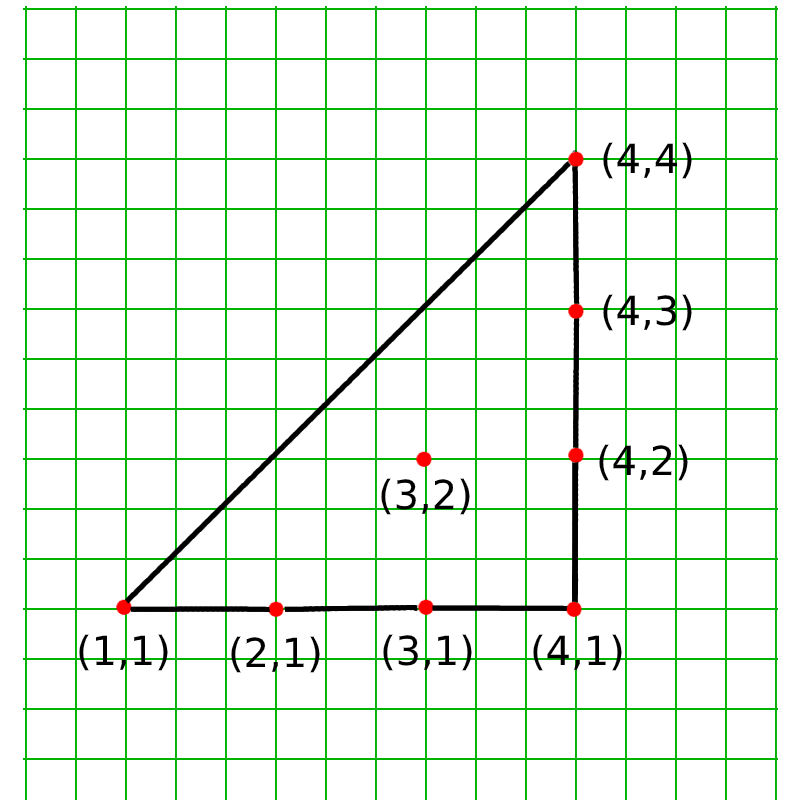# Check if the given point lies inside given N points of a Convex Polygon

• Difficulty Level : Medium
• Last Updated : 08 Jul, 2020

Given coordinates of the N points of a Convex Polygon. The task is to check if the given point (X, Y) lies inside the polygon.

Examples:

Input: N = 7, Points: {(1, 1), (2, 1), (3, 1), (4, 1), (4, 2), (4, 3), (4, 4)}, Query: X = 3, Y = 2
Below is the image of plotting of the given points:Output: YES

Input: N = 7, Points: {(1, 1), (2, 1), (3, 1), (4, 1), (4, 2), (4, 3), (4, 4)}, Query: X = 3, Y = 9
Output: NO

## Recommended: Please try your approach on {IDE} first, before moving on to the solution.

Approach: The idea is to use Graham Scan Algorithm to find if the given point lies inside the Convex Polygon or not. Below are some of the observations:

• Suppose the point (X, Y) is a point in the set of points of the convex polygon. If the Graham Scan Algorithm is used on this set of points, another set of points would be obtained, which makes up the Convex Hull.
• If the point (X, Y) lies inside the polygon, it won’t lie on the Convex Hull and hence won’t be present in the newly generated set of points of the Convex Hull.
• If the point (X, Y) lies outside the polygon, it will then lie on the Convex Hull formed and hence would be present in the newly generated set of points of the Convex Hull.

Below are the steps to solve the problem:

1. Sort the given points along with the query point in the increasing order of their abscissa values. If the abscissa values(x-coordinates) of any two points are the same, then sort them on the basis of their ordinate value.
2. Set the bottom-left point as the start point and top-right point as the end point of the convex hull.
3. Iterate over all the points and find out the points, forming the convex polygon, that lies in between the start and endpoints in the clockwise direction. Store these points in a vector.
4. Iterate over all the points and find out the points, forming the convex polygon, that lies in between the start and endpoints in the counter-clockwise direction. Store these points in the vector.
5. Check if the query point exists in the vector then the point lies outside the convex hull. So return “No”.
6. If the point doesn’t exist in the vector, then the point lies inside the convex hull print “Yes”.

Below is the implementation based on the above approach:

 `// C++ program for the above approach``#include ``using` `namespace` `std;`` ` `// Sorting Function to sort points``bool` `cmp(pair<``int``, ``int``>& a,``         ``pair<``int``, ``int``>& b)``{`` ` `    ``if` `(a.first == b.first)``        ``return` `a.second < b.second;``    ``return` `a.first < b.first;``}`` ` `// Function To Check Clockwise``// Orientation``int` `cw(pair<``int``, ``int``>& a,``       ``pair<``int``, ``int``>& b,``       ``pair<``int``, ``int``>& c)``{`` ` `    ``int` `p = a.first * (b.second - c.second)``            ``+ b.first * (c.second - a.second)``            ``+ c.first * (a.second - b.second);`` ` `    ``return` `p < 0ll;``}`` ` `// Function To Check Counter``// Clockwise Orientation``int` `ccw(pair<``int``, ``int``>& a,``        ``pair<``int``, ``int``>& b,``        ``pair<``int``, ``int``>& c)``{`` ` `    ``int` `p = a.first * (b.second - c.second)``            ``+ b.first * (c.second - a.second)``            ``+ c.first * (a.second - b.second);`` ` `    ``return` `p > 0ll;``}`` ` `// Graham Scan algorithm to find Convex``// Hull from given points``vector > convexHull(``    ``vector >& v)``{``    ``// Sort the points``    ``sort(v.begin(),``         ``v.end(), cmp);`` ` `    ``int` `n = v.size();``    ``if` `(n <= 3)``        ``return` `v;`` ` `    ``// Set starting and ending points as``    ``// left bottom and top right``    ``pair<``int``, ``int``> p1 = v;``    ``pair<``int``, ``int``> p2 = v[n - 1];`` ` `    ``// Vector to store points in``    ``// upper half and lower half``    ``vector > up, down;`` ` `    ``// Insert StartingEnding Points``    ``up.push_back(p1);``    ``down.push_back(p1);`` ` `    ``// Iterate over points``    ``for` `(``int` `i = 1; i < n; i++) {`` ` `        ``if` `(i == n - 1 || !ccw(p1, v[i], p2)) {`` ` `            ``while` `(up.size() > 1``                   ``&& ccw(up[up.size() - 2],``                          ``up[up.size() - 1],``                          ``v[i])) {`` ` `                ``// Exclude this point``                ``// if we can form better`` ` `                ``up.pop_back();``            ``}`` ` `            ``up.push_back(v[i]);``        ``}`` ` `        ``if` `(i == n - 1 || !cw(p1, v[i], p2)) {`` ` `            ``while` `(down.size() > 1``                   ``&& cw(down[down.size() - 2],``                         ``down[down.size() - 1],``                         ``v[i])) {`` ` `                ``// Exclude this point``                ``// if we can form better``                ``down.pop_back();``            ``}``            ``down.push_back(v[i]);``        ``}``    ``}`` ` `    ``// Combine upper and  lower half``    ``for` `(``int` `i = down.size() - 2;``         ``i > 0; i--)``        ``up.push_back(down[i]);`` ` `    ``// Remove duplicate points``    ``up.resize(unique(up.begin(),``                     ``up.end())``              ``- up.begin());`` ` `    ``// Return the points on Convex Hull``    ``return` `up;``}`` ` `// Function to find if point lies inside``// a convex polygon``bool` `isInside(vector > points,``              ``pair<``int``, ``int``> query)``{``    ``// Include the query point in the``    ``// polygon points``    ``points.push_back(query);`` ` `    ``// Form a convex hull from the points``    ``points = convexHull(points);`` ` `    ``// Iterate over the points``    ``// of convex hull``    ``for` `(``auto` `x : points) {`` ` `        ``// If the query point lies``        ``// on the convex hull``        ``// then it wasn't inside``        ``if` `(x == query)``            ``return` `false``;``    ``}`` ` `    ``// Otherwise it was Inside``    ``return` `true``;``}`` ` `// Driver Code``int` `main()``{`` ` `    ``// Points of the polygon``    ``// given in any order``    ``int` `n = 7;``    ``vector > points;`` ` `    ``points = { { 1, 1 }, { 2, 1 }, { 3, 1 },``               ``{ 4, 1 }, { 4, 2 }, { 4, 3 },``               ``{ 4, 4 } };`` ` `    ``// Query Points``    ``pair<``int``, ``int``> query = { 3, 2 };`` ` `    ``// Check if its inside``    ``if` `(isInside(points, query)) {``        ``cout << ``"YES"` `<< endl;``    ``}``    ``else` `{``        ``cout << ``"NO"` `<< endl;``    ``}`` ` `    ``return` `0;``}`

Output:

```YES
```

Time Complexity: O(N * log(N))
Auxiliary Space: O(N)

My Personal Notes arrow_drop_up